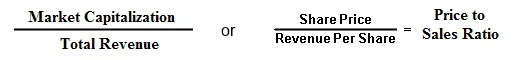Select Page

# The Price to Sales Ratio (P/S Ratio)The Price to Sales ratio (P/S ratio) is a financial ratio used among investors to determine the value of a company.

Similar to the P/E ratio, the P/S ratio gives investors an idea on how much they’re paying for a dollar of sales instead of a dollar of earnings. Essentially, the P/S number reveals the value placed on sales by the market.

The price to sales ratio can be a useful tool to use, instead of using the P/E ratio, especially if you’re examining start-ups, small-cap companies, and unprofitable companies with no earnings. If there are no earnings, you can’t calculate a P/E, but as long as a company has sales, or revenues, you can calculate a P/S.

The P/S ratio is often regarded by some stock market analysts as a better indicator of the growth potential of a company than the P/E ratio. This is because sales revenue is more difficult to manipulate by accounting tricks than earnings.

## How The Price to Sales Ratio is Calculated

The P/S ratio is calculated by dividing the current stock price by the sales per share. Another formula for the ratio is it can also be calculated by dividing the company’s market cap by total sales over the past 12 months.

Typically when calculated, the P/S uses the “trailing twelve months”, which is the reported sales for the previous four quarters, although longer time periods can be used.

Calculated as:
(Stock Price) / (Sales Per Share) = P/S or (Market Capitalization) / (Total Sales) = P/SFor example, a company named XYZ is trading at a share price of \$20 with sales per share of \$10. The price-to-sales ratio would be 2 (20 ÷ 10 = 2).

## What is a Good P/S ratio?

A low price-to-sales ratio, typically less than 1.0, is considered a good value as that means an investor is paying less for each sale.

The lower the P/S ratio, the better the value since the investor is paying less for each unit of sales. However, sales do not reveal the entire scope, as the company may be unprofitable with a low P/S ratio. Because of the limitations, this ratio is usually used only for unprofitable companies, since they don’t have a P/E ratio.

## More on The Price-to-Sales Ratio

As stated above the P/S ratio is a very handy ratio when examining companies with no earnings and therefore a P/E ratio can’t be determined. The P/S ratio is often used for start-ups, small-cap companies, and unprofitable companies with no earnings to help determine their growth potential and how the company is faring relative to its competitors.

In addition, there are companies in highly cyclical industries that experience cyclical lows in their earnings cycle and are unprofitable during that time, such as the auto industry. This does not mean auto stocks are worthless. In these cases, the P/S ratio comes in handy as you can simply apply this ratio instead of the P/E, which gives you an idea on how much you’re paying for a dollar of sales instead of a dollar of earnings.

The P/S ratio varies dramatically by industry, and therefore the P/S ratio should be used to compare a company’s value relative to its industry peers.

Despite its usefulness, though, the price to sales ratio should never be the only number to use. P/S ratios are usually used along with other metrics, such as the P/E ratio and among others that investors can use to evaluate stocks.